Author: Oscar Cronquist Article last updated on March 11, 2020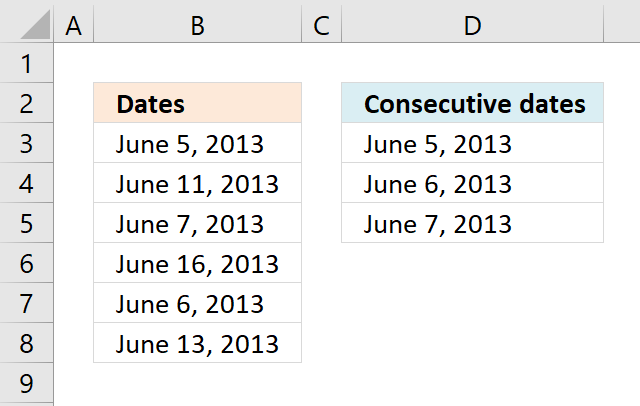The image above shows a formula in cell D3 that extract dates from column B. Column B contains dates in random order.

The condition that must be met is that at least two dates is exactly one day a part from each other. Duplicate dates are repeated in the returned date sequence.

Array formula in cell D3:

=IFERROR(SMALL(IF((COUNTIF(\$B\$3:\$B\$8, \$B\$3:\$B\$8+1) + COUNTIF(\$B\$3:\$B\$8, \$B\$3:\$B\$8-1))>0, \$B\$3:\$B\$8, ""),ROWS(\$A\$1:A1)), "")

This formula can be entered as a regular formula if you are an Excel 365 subscriber. Earlier versions must enter this formula as an array formula to make it work properly.

How to enter an array formula

1. Copy array formula above (Ctrl + c).
2. Doublepress with left mouse button on cell D3.
3. Paste formula (Ctrl  + v)
4. Press and hold CTRL + SHIFT simulateously.
5. Press Enter once.
6. Release all keys.

The formula now begins with and ends with a curly bracket, like this: {=array_formula}. Don't enter these characters yourself, they appear automatically if you followed the steps above.

How to copy array formula

1. Select cell D3.
2. Copy cell (Ctrl + c).
3. Select cell range D4:D5.
4. Paste (Ctrl + v).

The relative cell refences in the formula changes automatically when you copy the cell (not the formula) and paste to cells below.

### Explaining the formula in cell D3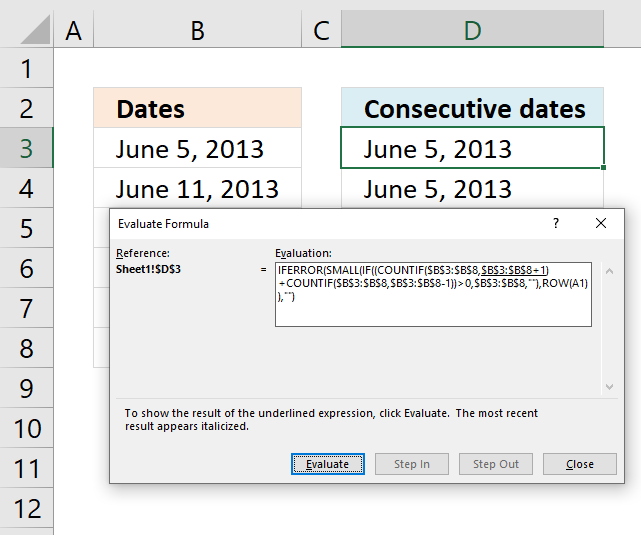To understand formulas in greater detail I recommend you use the "Evaluate Formula" tool. Go to tab "Formulas" on the ribbon. Press with left mouse button on the "Evaluate Formula" button and a dialog box appears.

Press with left mouse button on the "Evaluate" button on the dialog box to show the next calculation step by step. Press with left mouse button on "Close" button to dismiss the dialog box.

#### Step 1 - Find dates that have a consecutive date except for the last consecutive date

The COUNTIF function counts cells based on a condition, however, we are going to use multiple conditions. The COUNTIF function will, in this case, return an array with values equal to the number of conditions. Their position in the array matches the position of each condition.

COUNTIF(rangecriteria)

The formula is working with dates so I now need to explain how Excel handles dates. Excel dates are actually regular numbers, number 1 is equal to 1/1/1900 and number 36526 is 1/1/2000. There are 36525 days between 1/1/2000 and 1/1/1900. Excel basically formats cells containing numbers as dates.

You can check this yourself, type 1/1/1900 in a cell. Select the cell and press CTRL + 1 to open the "Format Cells" dialog box. Press with left mouse button on "General" and press the OK button. The selected cell now shows 1.

COUNTIF(\$B\$3:\$B\$8,\$B\$3:\$B\$8+1)

becomes

COUNTIF({41430; 41436; 41432; 41441; 41431; 41438}, {41430; 41436; 41432; 41441; 41431; 41438}+1)

becomes

COUNTIF({41430; 41436; 41432; 41441; 41431; 41438}, {41431; 41437; 41433; 41442; 41432; 41439})

and returns {1; 0; 0; 0; 1; 0}. This array tells us which dates have a consecutive date, however it will not tell us which date is the last consecutive date. The next step will take care of that.

#### Step 2 - Find dates that have a consecutive date except the first consecutive date

COUNTIF(\$B\$3:\$B\$8,\$B\$3:\$B\$8-1)

becomes

COUNTIF({41430; 41436; 41432; 41441; 41431; 41438}, {41430; 41436; 41432; 41441; 41431; 41438}-1)

becomes

COUNTIF({41430; 41436; 41432; 41441; 41431; 41438}, {41429; 41435; 41431; 41440; 41430; 41437})

and returns {0; 0; 1; 0; 1; 0}. This arraray shows us which dates are the last consective date for any given sequence.

#### Step 3 - Add arrays

To identify all consecutive dates we must add both arrays meaning we are applying OR logic.

COUNTIF(\$B\$3:\$B\$8,\$B\$3:\$B\$8+1)+COUNTIF(\$B\$3:\$B\$8,\$B\$3:\$B\$8-1)

becomes

{1;0;0;0;1;0} + {0;0;1;0;1;0} and returns {1;0;1;0;2;0}.

#### Step 4  - Find values in array larger than 0 (zero)

This step is actually not needed, the IF function will in the next step handle any number as the boolean value TRUE and 0 (zeros) as FALSE. There is really no need to convert the array to their boolean counterparts.

(COUNTIF(\$B\$3:\$B\$8,\$B\$3:\$B\$8+1)+COUNTIF(\$B\$3:\$B\$8,\$B\$3:\$B\$8-1))>0

becomes

{1;0;1;0;2;0}>0

and returns {TRUE; FALSE; TRUE; FALSE; TRUE; FALSE}.

#### Step 5 - Filter consecutive dates

The IF function returns the corresponding date if the logical expression returns TRUE and a blank if FALSE.

IF((COUNTIF(\$B\$3:\$B\$8, \$B\$3:\$B\$8+1) + COUNTIF(\$B\$3:\$B\$8, \$B\$3:\$B\$8-1))>0, \$B\$3:\$B\$8, "")

becomes

IF({TRUE; FALSE; TRUE; FALSE; TRUE; FALSE}, \$B\$3:\$B\$8, "")

becomes

IF({TRUE; FALSE; TRUE; FALSE; TRUE; FALSE}, {41430; 41436; 41432; 41441; 41431; 41438}, "")

and returns {41430; ""; 41432; ""; 41431; ""}.

#### Step 6 - Find the k-th smallest date

The SMALL function extracts the k-th smallest number using the ROWS function and absolute and relative cell references in order to return values in a cell each.

SMALL(IF((COUNTIF(\$B\$3:\$B\$8, \$B\$3:\$B\$8+1) + COUNTIF(\$B\$3:\$B\$8, \$B\$3:\$B\$8-1))>0, \$B\$3:\$B\$8, ""),ROWS(\$A\$1:A1))

becomes

SMALL({41430; ""; 41432; ""; 41431; ""}, ROWS(\$A\$1:A1))

becomes

SMALL({41430; ""; 41432; ""; 41431; ""}, 1)

and returns 41430 (June 5, 2013) in cell D3. The SMALL function will sort the output dates from smallest to largest.

### Conditional formatting consecutive dates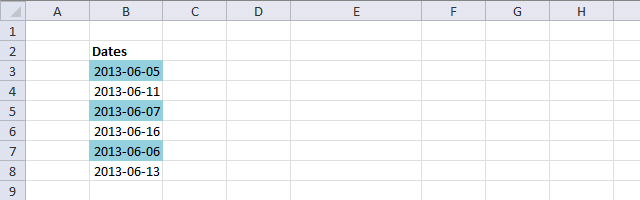You can also highlight consecutive dates using Conditional formatting.

Conditional formatting formula:

=(COUNTIF(\$B\$3:\$B\$8,B3+1)+COUNTIF(\$B\$3:\$B\$8,B3-1))>0

#### How to apply conditional formatting

1. Select cell range B3:B8.
2. Go to "Home" tab on the ribbon.
3. Press with left mouse button on the "Conditional formatting" button.
4. Press with left mouse button on "New Rule...".
5. Press with left mouse button on "Use a formula to determine which cells to format".
6. Format values where this formula is TRUE:
=(COUNTIF(\$B\$3:\$B\$8,B3+1)+COUNTIF(\$B\$3:\$B\$8,B3-1))>0
7. Press with left mouse button on "Format.." button.
8. Press with mouse on the "Fill" tab to select it.
9. Pick a color.
10. Press with left mouse button on OK button.
11. Press with left mouse button on OK button.

### Find consecutive numbers

Since dates are numbers in excel you can also use the array formula to identify consecutive numbers.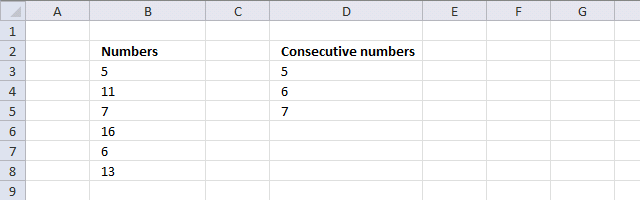### Find non-consecutive dates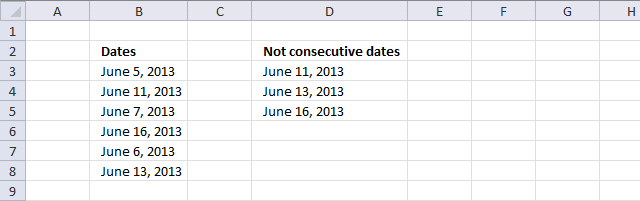Array formula in cell D3:

=IFERROR(SMALL(IF((COUNTIF(\$B\$3:\$B\$8, \$B\$3:\$B\$8+1)+COUNTIF(\$B\$3:\$B\$8, \$B\$3:\$B\$8-1))=0, \$B\$3:\$B\$8, ""), ROW(A1)), "")

### Get the Excel fileConsecutive-dates.xlsx